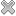# Courses / Module

Toggle Print

##LINEAR MODELS 1 (R)

Module code: ST863
Credits: 5
Semester: 1
Department: MATHEMATICS AND STATISTICS
International:Overview

Module Objective: To introduce students to Linear Models. Scatterplots and regression. Simple linear regression: estimation, parameter inference, prediction, analysis of variance, R2, F-test, correlation, residual plots, assessing normality. The multiple regression model and its applications. Matrix notation, mean vectors and covariance matrices. Least-squares estimation. The multivariate normal distribution. Hypothesis testing and confidence intervals, prediction. Analysis of variance, R2, sequential sums of squares, general F-tests. Added variable plots. Model checking via testing for lack of fit and residual plots. Regression diagnostics: residuals, leverage, outliers, influence and Cook's Distance.

Lectures and tutorials as for ST463.Learning OutcomesTeaching & Learning methodsAssessmentRepeat optionsPre-RequisitesTimetableV5.2.0 - Powered by MDAL Framework © 2019